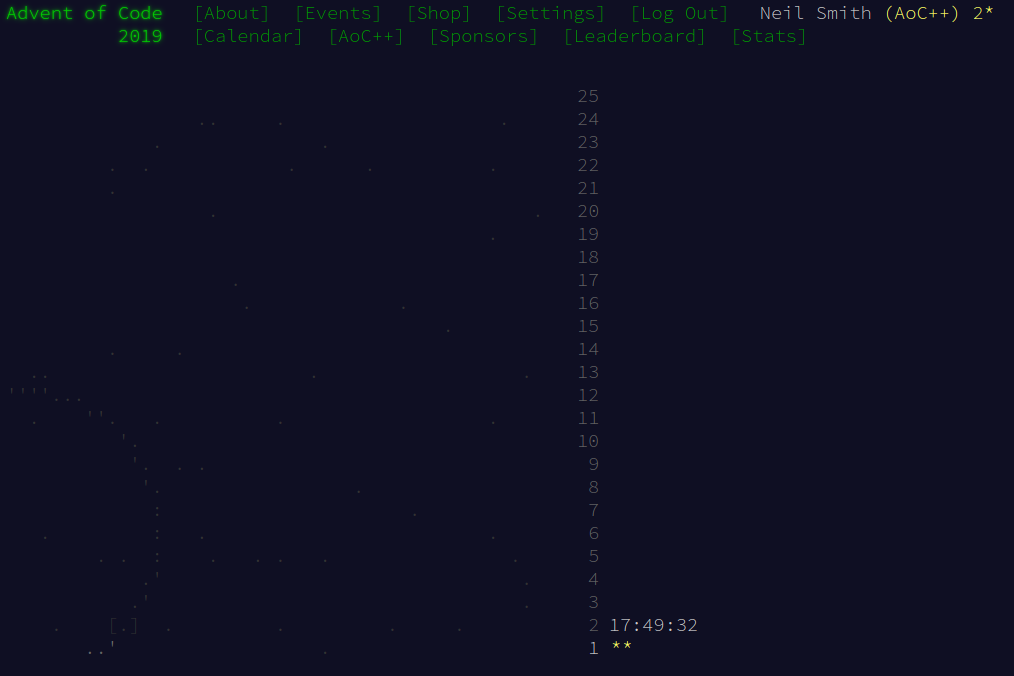December 1, 2019

# Advent of Code 2019 day 1

Beginner-friendly description of solving Day 1.This year, I thought I'd write up my solutions to the Advent of Code series of challenges. As with the previous few years, I'll be using Haskell to solve the challenges, as a vehicle to exercise my functional programming skills. In particular, I'm hoping to get more used to using some of the more advanced concepts, such as Monoids, Foldable, and Traversable.

I'm keeping my code on Github for people to look at. But without further ado, here's…

## Day 1

For those following along, the code is on Github.

The problem involves reading a data file, then processing the data to give the answer.

For reading the data, I tend to use the Megaparsec library. It tends to mean you write much more understandable parsers than the sort of line-noise you get with regular-expression based parsing. Beyond some boilerplate, the guts of the parser is simply the line:

moduleP = many integer


which says the modules file simply comprises many integers. That's pretty readable, but it's a very simple data file. I'm sure the parsing side will become more interesting in later challenges.

### Processing the data

The basic idea is to process each element of the data file and then add up all the processed values. Something that Haskell (and other functional languages) is good at is capturing these patterns of processing and reifying them, to be implemented as higher-order functions. The "process each element of a collection" is known as a map, and combining all the values in a collection is known as a fold. (In fact, Haskell has a pre-made fold that adds up all the numbers in a list; it's called sum.) That suggests what the solution should look like:

solution1modules = sum (map fuelRequired modules)


leaving us to write the fuelRequired function to calculate the fuel requirements for each module:

fuelRequired module = (module div 3) - 2


We can tidy up the solution a bit with the $ operator, which allows us to get rid of the brackets: solution1 modules = sum$ map fuelRequired modules


We can go further, using partial application to express the solution function as purely the composition of other functions:

solution1 = sum . map fuelRequired


(This is also called point-free style of programming.)

### Part 2

Part 2 adds some more complexity by making the fuel requirements harder to calculate, by requiring a repeated calculation. In an imperative language, that's something we'd probably build with a while loop. In a functional language, we could write a recursive function to do the repeated calculations. But the general idea of "take an input, calculate a result; take that result, calculate the next result; take that result, calculate the next result; …" is common. Much like map and fold represent common patterns of calculation, the "repeated calculation" idea is implemented as iterate.

iterate takes a function and an initial value, and generates the infinite list that comes from repeatedly applying the function to the most recent result. For instance, the function

iterate (+1) 0


will generate the infinite list [0, 1, 2, 3, 4, …]. In this problem, the repeated fuel calculations can be performed by:

fuelForFuel module = sum $iterate fuelRequired module  ("Calculate the fuel required for the module, then calculate the fuel required for the fuel, then the fuel required for that fuel, and so on. Then add up all the fuel requirements.") But this doesn't quite work. There are two problems. First is that iterate returns a list that contains the intial value. We don't want that, but we can get rid of it by dropping the first element: fuelForFuel module = sum$ drop 1 $iterate fuelRequired module  The larger problem is that this calculation will never terminate: iterate will generate an infinite list of results, and sum will wait for the list to end before it returns its value. We get around that by only taking fuel requirements while they're positive, using takeWhile. fuelForFuel module = sum$ takeWhile (> 0) $drop 1$ iterate fuelRequired module


As before, we can use partial application to express the whole thing in point-free style:

solution2 = sum . map fuelForFuel
fuelForFuel = sum . takeWhile (> 0) . drop 1 . iterate fuelRequired


And that's it!